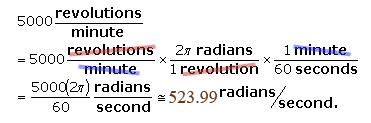SEARCH HOMEMath Central Quandaries & QueriesQuestion from virginia, a student: The engine of a sports car rotates at 5,000 revolutions per minute (rpm). calculate the angular speed of the engine in radians per second. use 2n radians = 1 revolutionHi Virginia.

I think you were trying to write 2π radians = 1 revolution.

Angular speed is the amount of angle through which the engine turns in a given amount of time.

In 1 minute, the engine turns around 5000 times, so it has gone 5000 revolutions. Since there are 2π radians in each revolution and 60 seconds in each minute, this is 5000×2π / 60 radians per second.

In unit evaluation method, you would write it this way:Hope this helps,
Stephen La Rocque.Math Central is supported by the University of Regina and The Pacific Institute for the Mathematical Sciences.